﻿ Electrical Conduction and Dielectric Relaxation in Selenium Films Doped with Dysprosium Rare EarthAmerican Journal of Condensed Matter Physics

p-ISSN: 2163-1115    e-ISSN: 2163-1123

2017;  7(2): 41-49

doi:10.5923/j.ajcmp.20170702.02### Electrical Conduction and Dielectric Relaxation in Selenium Films Doped with Dysprosium Rare Earth

Fathy A. Abdel-Wahab, Heba Abdel Maksoud

Physics Department, Faculty of Science, Ain Shams University, Abbassia, Cairo, Egypt

Correspondence to: Fathy A. Abdel-Wahab, Physics Department, Faculty of Science, Ain Shams University, Abbassia, Cairo, Egypt.
 Email: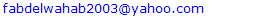Abstract

Selenium (Se) films implanted with Dysprosium (Dy) atoms are prepared using thermal evaporation technique. The temperature dependence of ac conductivity measured in frequency range 50 Hz-80 KHz is found to be proportional to ωs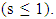This proportionality is reasonably well interpreted, in the low temperature region, by correlated barrier hopping (CBH), of bi-polaron between both random and paired defect states. In the same time the high temperature part the ac conductivity is well fitted using Mayer-Neldel rule. Furthermore, the dielectric relaxation times of the doped a-Se films are determined from the dependence of the loss factor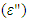on the applied frequency relations. The Cole-Cole diagrams have been presented and are used to determine the values of the static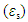and optical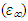dielectric constants of a-Se doped with Dy atoms.

Keywords: Amorphous Selenium, Doped chalcogenides, Rare earths, ac conductivity, Dielectric relaxation and loss, Cole-Cole diagram

Cite this paper: Fathy A. Abdel-Wahab, Heba Abdel Maksoud, Electrical Conduction and Dielectric Relaxation in Selenium Films Doped with Dysprosium Rare Earth, American Journal of Condensed Matter Physics, Vol. 7 No. 2, 2017, pp. 41-49. doi: 10.5923/j.ajcmp.20170702.02.

### 1. Introduction

Rare-earth (RE)-doped glass fiber amplifiers operating at a 1.3 μm wavelength band have received extensive attention due to the zero dispersion of the silica fiber glass in the 1.3 μm-wavelength region, and most installed fibers worldwide are optimized at this wavelength. [1-4]. In contrast, Dysprosium rare earth atoms, Dy which have an active unfilled f shells in its electronic configuration ([Xe] 4f104s2), can provide 1.3 μm emission due to the 6F11/2, 6H9/26H15/2 transition . In addition, Dy has a good absorption band at approximately 800 nm, at which level a cheap commercial laser diode could be used for excitation.
On the other hand, amorphous Selenium (a-Se) is characterized by existence of localized states in its mobility gap. These states are created due to presence of defects and absence of long range order [6-8]. The structural disorder made a-Se and its alloys to have a high optical transparency in the infra-red (IR) spectral regions up to 10 μm. Besides, it has large refractive index, thermal stability and high degree of covalent bonding. Furthermore, due to high rare earth solubility, high emission quantum efficiency  and the low phonon energy (~ 250 cm-1) of amorphous selenides compared with fluorides (~ 550 cm-1), or oxide glasses (~ 1100 cm-1)  it could be used as a host medium for Dy to enhance its mid-IR laser emission. It should be noted that the low phonon energy of a-se decreases the multi phonon relaxation which enables an active transitions between rare earth atom levels in the middle IR spectral region. The optical excitation and emission of Dy is very sensitive to the surrounding media considerations such as dielectric parameters which plays an important role to control the relaxation processes and consequently the performance of the laser emission of Dy .
In the present work, the dependence of ac conductivity on the applied frequency and temperature are analyzed with reference to the current conduction models. The analysis showed that the correlated barrier hopping over barriers (CBH) is the most probable model to describe the a.c. conduction mechanism in the low temperature range. Furthermore, the modified CBH with Mayer-Neldel rule is well fitted with the ac conduction in the high temperature range. The dependence of the loss factor (ε") on the applied frequency and Cole-Cole diagrams showed that the dielectric relaxation times for Se-Se and Se-Dy dipoles are 2.46x10-5 and 1.27x10-4 s in sequence.

### 2. Experimental

Bulk Selenium sample of weight 3g and doped with 0.008 (at. %) of dysprosium (Dy) is prepared by mixing appropriate quantities of Se and Dy, of purity 5N, in evacuated pre-cleaned silica tube sealed at 10-5 Torr. The synthesis of the mixture was carried out by heating the ampoule in an electric furnace to 400°C and keeping it at this temperature for 3 hrs. Then, the temperature (T) was gradually raised by a rate of ~ 200°C/h to 900°C and kept again at that temperature for another 9 hrs. Finally, the ampoule is left to cool down to room temperature (RT=25°C).
The obtained bulk ingots were used as source materials to prepare thin film samples by the thermal evaporation technique using vacuum coating unit type Edwards E306 A. The base pressure of the vacuum system was 1.5x10-6 Torr. After evaporation, the thickness of the fresh films was accurately determined by an optical interference method and is found to be 750 nm. The structure of the obtained films are studied using x-ray diffraction computerized system (model: Philips EXPERT-MPDUG PW-3040 diffractometer with Cu Kα radiation source) in the 2θ range 0–90°. For electrical characterization, sandwich structure devices consisting of Au/Se:Dy/Au were prepared in situ by thermal sublimation under high vacuum (~10-5 Torr). A programmable voltage–current source (type Keithley 228 A) was used as a source of applied voltage and a Keithley-616 digital electrometer was used to measure the output current. The electrical conductivity measurements were done under vacuum and steady state conditions, using lock-in amplifier model Oxford-DN 1714 in the temperature range 312-82 K and frequency range 50 Hz–80 kHz in the dark conditions.

### 3. Results and Discussion

The recorded XRD patterns for the studied as-prepared a-Se, previous work of SeSm0.008 films  and Dy doped Se (present work) samples are shown in figure (1). In this figure, the XRD pattern of the fresh Se films reflects its amorphous nature. Furthermore, the doped Se film with 0.008 at.% Sm  reflects an amorphous matrix embedded with some crystalline zones. These zones appear throughout two characteristic selenium lines lying in the region of the main diffraction hump covering roughly the range of 23-32 in 2θ° angular units. Disappearance of such diffraction hump in case of Se doped with 0.008 at. % Dy (present work) mean the growth of crystalline phase on the expense of amorphous state. This crystalline phase consists of mixed phases of elemental Se (100), (101), (012), (110), (111), (022) elemental Dy (102), (110) and SeDy with tetragonal (002), (003), (112) and orthorhombic (603), (116), (117) structures as shown in Fig. (1).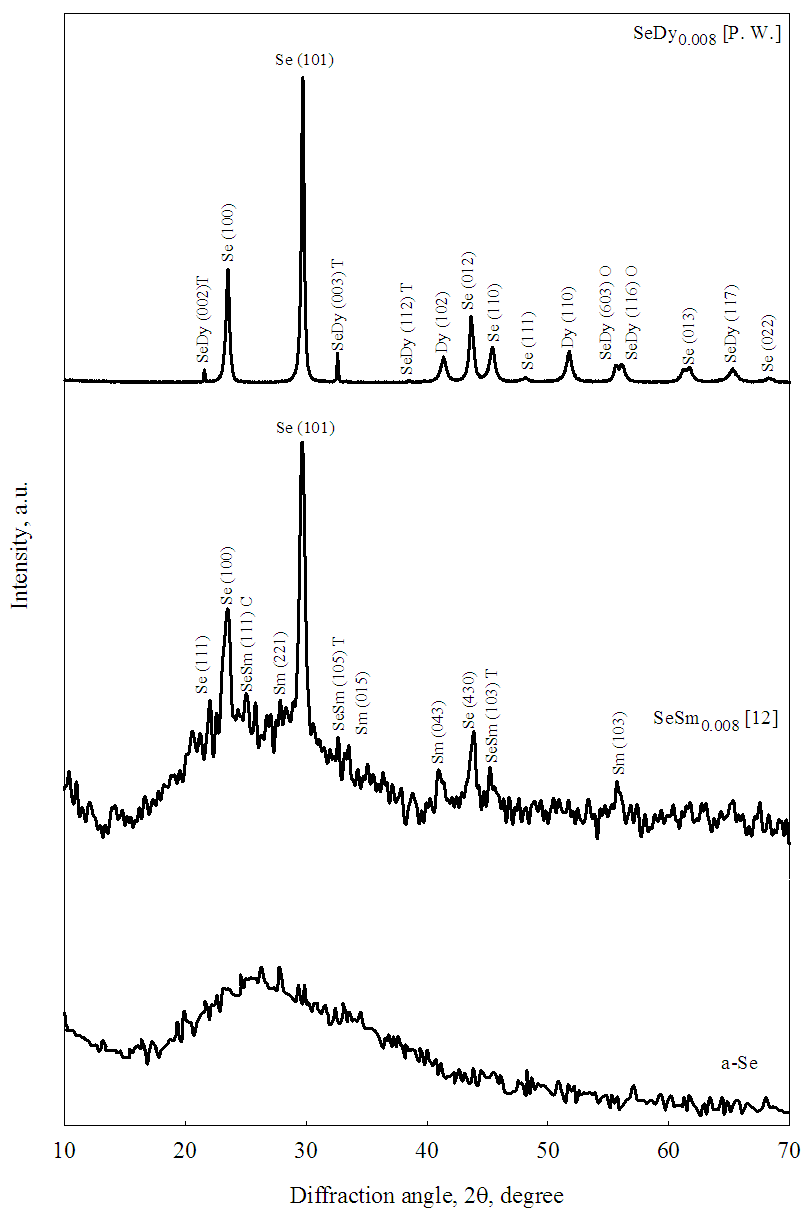Figure (1). XRD pattern for a-Se, previous work of SeSm0.008  and the present work [P. W.] of SeDy0.008 thin films. It should be noted that the diffraction pattern of SeSm0.008  is added to the figure for the sake of comparison

#### 3.1. Electrical Conduction

##### 3.1.1. ac Conduction
The temperature dependence of ac conductivity (σac) of the investigated samples at different values of frequency is shown in Fig. (2). For clarification purpose, not all the recorded frequency data points are represented in the figure. In this figure, the ac conductivity shows a frequency dependence which becomes more pronounced at higher frequencies. On the other hand, and in low temperature range (82-152 K) the conductivity increase slightly less than linear against temperature and tends to become a temperature dependent at higher temperatures (152-312 K) in the studied frequency range. The graphical relation between the applied frequency and ac conductivity (σac) at different temperatures is shown in Fig. (3). This figure illustrates that σac depends on temperature nearly in the whole studied frequency range. The enhance of ac conductivity at any frequency and temperature may be explained as follows: the Dy atoms enters the structural network of a-Se with coordination number of 7-8 bonds to Se with bond length from 2.92 to 2.97 °A . This means that a redistribution of electronic charges takes place which built up a space charge region around Dy atom and the surrounding Se atoms resulting an increase in σac.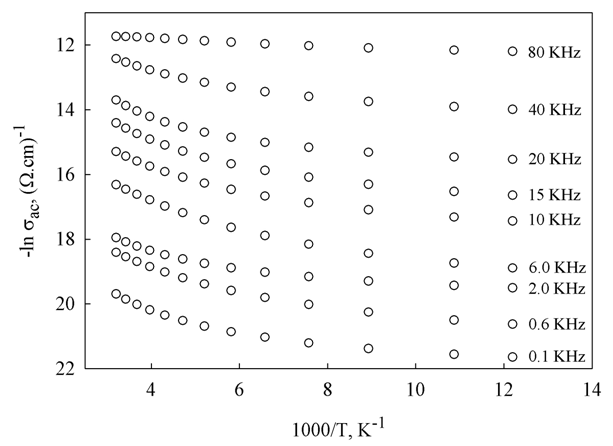Figure (2). Temperature dependence of ac conductivity for SeDy0.008 thin films measured at different frequencies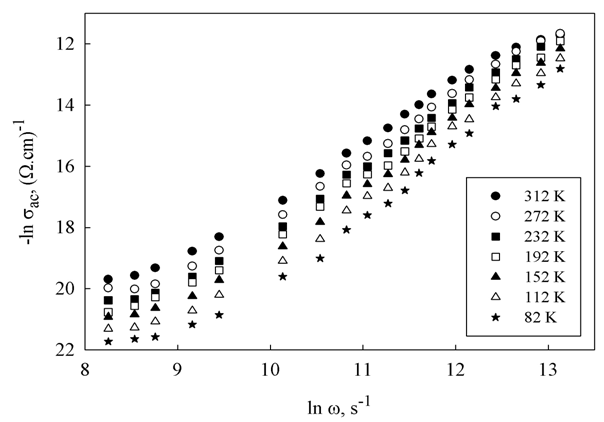Figure (3). ac conductivity dependence on frequency for the studied SeDy0.008 thin films measured at different temperatures
It is known that dc and ac conductivities are related to each other by an empirical relation of the form : σ(ω,T) = σdc+σac, where ω is the angular frequency of the applied alternating electric field. The a.c. conductivity is generally analyzed by considering a power law: σac(ω)=Aωs where A is a temperature dependent pre-exponential factor, s is the power exponent,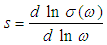which is used to determine the a.c. conduction mechanism . The temperature dependence of the exponent s calculated using Fig. (3) is shown in Fig. (4). This figure illustrates that the highest value of s is obtained at a lower measured temperature and decreases gradually with increasing temperature to reach a minimum value at T = 312 K.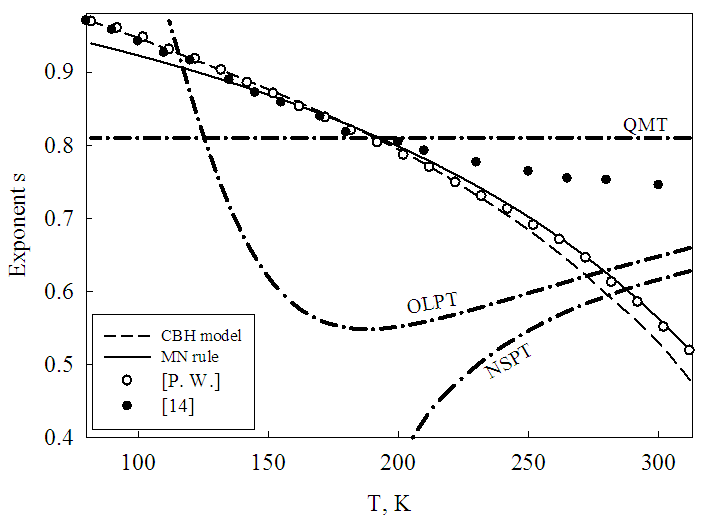Figure (4). Temperature dependence of the frequency exponent s (open circles) for the studied thin film samples [P. W.] obtained from slopes of Fig. (3). The dash-dotted curves represent the values calculated using the models: QMT , NSPT , OLPT  while the solid line represents the MN rule (Eq. (6)). The dashed curve represents the CBH model (Eq. (1)). The values of s calculated for Bismuth-Vanadate glassy semiconductor  are inserted in the figure for the sake of comparison
In the literature, various models have been proposed to explain the behavior of s which represents the exponent of Jonscher universal low for ac conductivity for semiconductors [6, 16]. These models differentiate between tunneling and hopping mechanisms. The tunneling which describes the overlap of the exponentially decaying wave function of the electrons due to its transition between two neighboring sites at a separation R are explained by simple quantum mechanical tunneling (QMT) , non-overlapping small polarons (NSPT)  and overlapping large polaron tunneling (OLPT)  models. On the other hand, "hopping" which is represented by correlated barrier (CBH) model, Elliott [18, 19] stated that for neighboring sites at a separation R, the Coulomb wells overlap, resulting in a lowering of the effective barrier height from WM to a value W. Here the index s is evaluated as :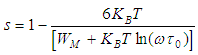(1)
Thus, in the CBH model a temperature dependent exponent s is predicted, with s increasing towards unity as T → 0.0 K. More details about these conduction models are given elsewhere [14, 20].
Taking into consideration the studied temperature range and at typical values of the parameters τ0=10-12 s, ω =104 s-1, the theoretical trend of the function s = f(T) for the QMT , NSPT , OLPT  and CBH models (Eq. (1)) is shown in Fig. (4) together with the experimental data of the present work [P. W.]. The similarity between the experimental s and CBH model reveal that CBH is fairly good to describe the ac conduction mechanism for the studied samples in the low temperature range (82-187 K) and deviate from experimental data trends in the higher temperature region. The coincidence in s in the low temperature range is also observed for other compositions such as Bismuth-Vanadate glassy semiconductor  as shown in Fig. (4).
The frequency dependent conductivity in the CBH model  is given by the following equation: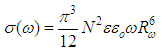(2)
where εεo is the dielectric permittivity of the material, N is the concentration of pair sites and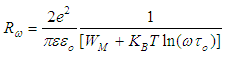(3)
To facilitate the obtained data shown in Fig. (4) the experimental σac data in Fig. (2) are fitted using equations (2) and (3) as shown in Fig. (5). Values of the parameters WM, τo, ε, and N used in the fitting procedure are 2.1 eV, 4x10-13 s, 3.5 and 1.4x1021 cm-3 in sequence for the investigated films. It should be noted that values of the dielectric constant, ε, of the studied thin films are obtained from the Cole–Cole diagram given in Section (3.2). In Fig. (5), it is observed that in the studied frequency range the fitting using CBH model (dashed lines) appears to be reasonable in the low temperature range and deviates from the experimental σac trend in the high temperature region as deduced from Fig. (4) of the present work. On the other hand, fitting using MN rule ﬁts well in the high temperature region and deviates from this trend as the temperature decreases with respect to the ﬁtting by the CBH model (Eq. (2)).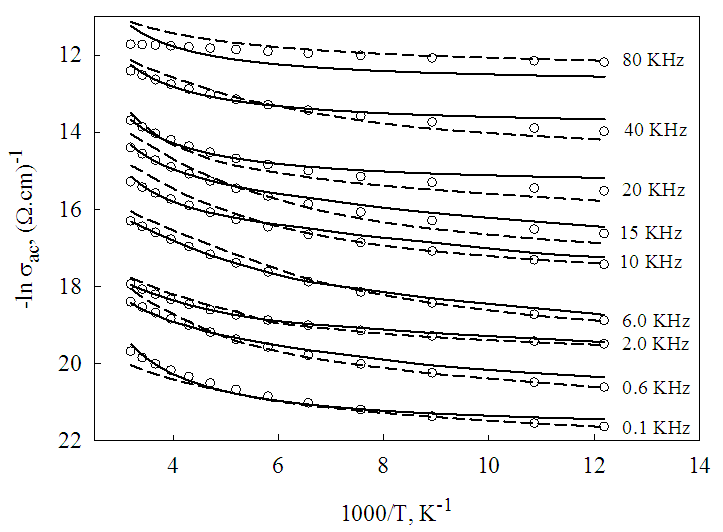Figure (5). Temperature dependence of ac conductivities measured at different frequencies for the studied thin films. The open circle symbols correspond to experimental data. The dashed curves represent the fitting of ac conductivity using the CBH model (Eq. (2)) while the solid curves are the fitting using the MN rule (Eq. (5))
##### 3.1.2. Modification of CBH Model using MN Rule
It is known that the CBH model explains the variation of σac(ω) with temperature at relatively low temperature and does not predict the strong temperature dependence at high temperatures [21, 22]. Mayer-Neldel (MN)  has improved the CBH model in order to account for the ac conductivity, σac, and for the frequency exponent s in the high temperature range. In the case of thermally activated processes, the MN rule states that the electric conductivity can be expressed by Arrhenius relation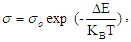the dependence of the pre-exponential factor,and activation energy,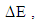in its logarithmic form is given by: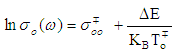(4)
where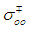and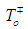are constants and refer to the normal and inverted MN rule in sequence. To apply MN rule on the studied samples, obtain the slopes and pre-exponential factors of the curves in Fig. (2) in the high temperature region. Plot the graphical representation of the pre-exponential factor,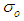and activation energy, , is shown in Fig. (6). The data points shown in Fig. (6) obey Eq. (4). Also, in this figure, it is observed that the experimental data at low frequencies obey the normal MN rule, while the high frequency data are found to obey the inverted MN rule. Obtained values of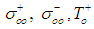and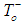for the studied samples are 5.7x10-8 (Ω.cm)-1, 6x10-6 (Ω.cm)-1, 845 K and 580 K respectively.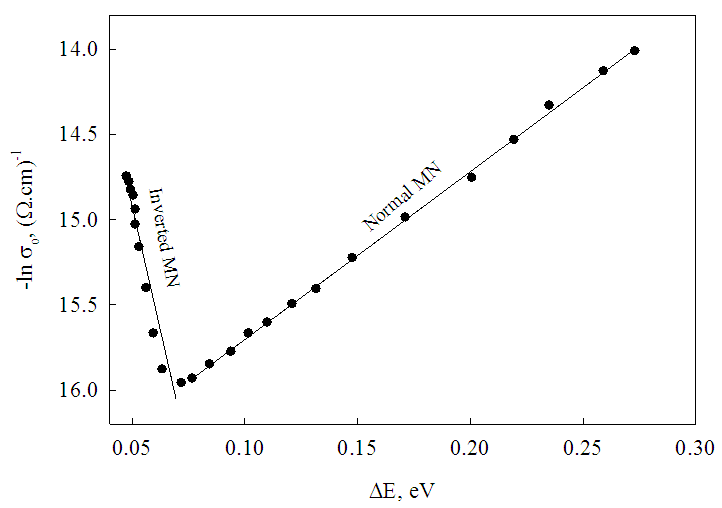Figure (6). Normal and inverted Meyer–Neldel plots for the studied samples. The symbols represent the experimental data and the straight lines are the best fit to the experimental data using least squares fits
The expression of ac conductivity of the CBH model based on the MN rule can be given as :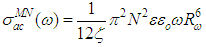(5)
where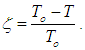While the power exponent s obeys the following relation: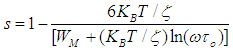(6)
It should be noted that Eqs. (5) and (6) differ from Eqs. (1) and (2) by the presence of MN rule factor (ζ) in the former equations. It is instructive to see the effect of the ζ factor (i.e., To) on the ac conductivity and the frequency exponent s. These have been calculated numerically using values of the parameters characterizing samples under study. The variation of exponent s with temperature as calculated using the MN rule (Eq. (6)) is represented in Fig. (4). However, it can be clearly seen from this ﬁgure that the trend of s is to decrease from nearly unity with increasing temperature and does not ﬁt with the experimental data of s.
This trend could be explained as follows:
When an atom is doped in a host material such as the present case of doping selenium with Dy atom an electron phonon coupling takes place between an electron of Dy atom and phonon which results from the oscillations of host lattice. These coupling has a quantized energy in a quantized states. These states will lie at the bottom of conduction band and top of valence bands and are used for relaxation of the excited electron in conduction band and its corresponding hole in the valence band [25, 26].
At high temperatures, the lattice oscillations increase which affect two main factors 1) increase the density of the electron-phonon coupling which in turn speeds up the relaxation process. 2) the value of the dielectric constant (ε, (equations (2 and 5)) which is directly proportional to the oscillations of the host lattice. These two factors increase the conductivity of the system. By lowering the temperature the lattice oscillation decreases which slow down the relaxation process and in the same time it decrease the dielectric electric constant (ε) and led to a decrease in the conductivity of the system.
The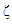term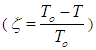which differentiate between the conductivity of CBH model (eq. (2)) and MN rule (eq. (5)) has a big role in the conductivity of the system. As temperature increase,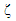decrease which increase the conductivity term in MN rule over the CBH model. On the other hand, at low temperature the value of ζ increase leading to a decrease of the conductivity value of CBH model over the MN rule.

#### 3.2. Dielectric Properties

Figure (7) shows the dependence of both real, ε', and imaginary, ε" parts of the complex dielectric constant on the applied angular frequency for the investigated samples in the studied temperature range. In this ﬁgure the real part of the dielectric constant, ε', changes only slowly with frequency in the low frequency range (400 Hz - 3.0 kHz) and decreases more rapidly at higher frequencies (3.0 - 80 kHz). Furthermore, in this figure it is observed that loss factor ε" exhibits two relaxation peaks in the low and high frequency regions. Appearance of these two peaks could be explained as follows: According to oscillator model of Lorentz  the natural resonant frequency of dipole is determined by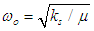where μ is the reduced mass of the two oscillators of the dipole and ks is the restoring force of the spring that connects them. It is known that doping of Selenium with Dy ions creates a Se-Dy bonds besides the Se-Se bonds of a-Se. Taking into consideration the atomic masses of Se (78.96) and Dy (162.5) we can conclude that for Se-Dy bonds, ωo will shift towards lower frequencies with respect to the Se-Se bonds and causes the formation of the peak at lower frequency as shown in Fig. (7). According to this model, the transition time τ of the two peaks (τ1 and τ2) could be calculated as the reciprocal of the angular frequency at the top of the loss peak. The calculated average values of τ1 and τ2 for the investigated samples calculated from ε"=f(ω) are 1.72x10-4 s and 2.31x10-5 s respectively.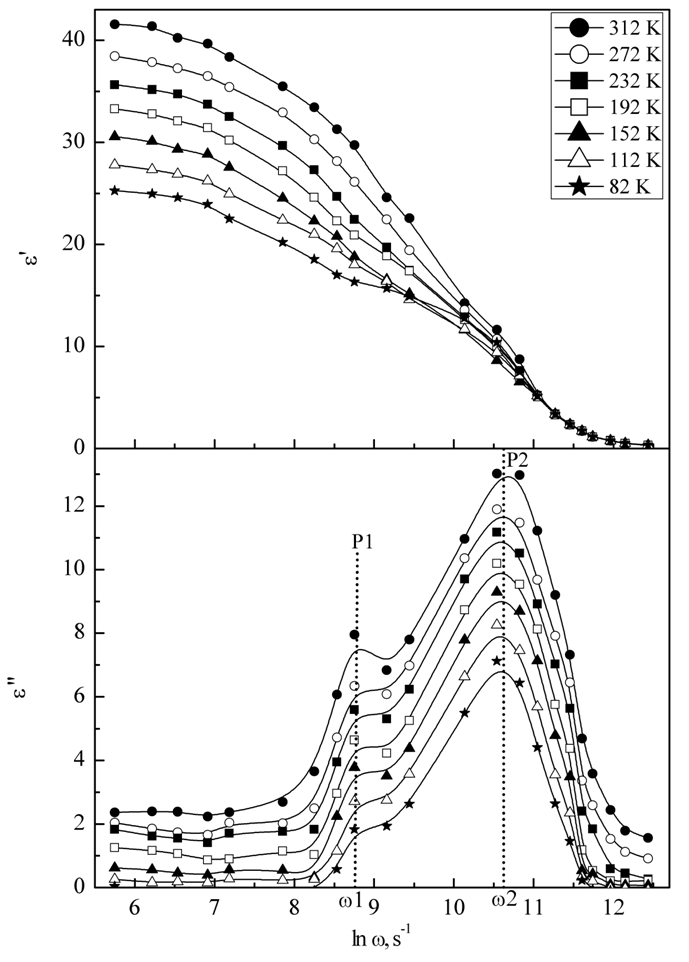Figure (7). Dependence of the real, ε', and imaginary, ε" parts of the complex dielectric constant on the applied angular frequency for the investigated samples in the studied temperature range
As is well known, the dependence of dielectric constants of a solid on the applied frequency decrease from a static value εs at low frequency to a smaller value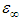at higher frequencies. The difference between values of εs and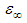is attributed to the polarization of the oscillating dipole . In this case, the relation between εs,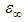and ε* is given by Cole and Cole  as: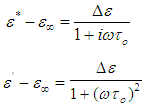(7)
and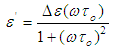(8)
where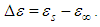The dependence of ε" on ε' is obtained by applying the Cole–Cole model for the investigated samples in the studied temperature range and is shown in Fig. (8). In this figure, the symbols represents calculated data with the solid line showing the best fit to the calculated data using Eq. (7). After extrapolating the fitted values to the real axis (ε'-axis), the plot shows that, for lower frequency the experimental points deviate from the dashed semicircles giving different values of the dielectric constant εs in the studied temperature range as shown in the inset of Fig. (8). In this inset of the figure it is clear that, in the low temperature region up to 200 K static dielectric constant, εs, changes by ratio ~ 26% against temperature while at higher temperatures (T > 200 K) the dependence becomes more pronounced and εs increase by 58%. The direct proportion between εs and temperature is due to the dependence of the static dielectric constant on the lattice oscillations of the host material . On the other hand in Fig. (8), at higher frequencies, all the data at different temperatures converge in one point that represents a unique value of optical dielectric constant,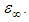The calculated values of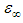for samples under study are 1.82. It should be noted that presence of two dielectric constants εs and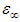suggest the presence of two relaxation processes with time constants whose values are rather close to each other.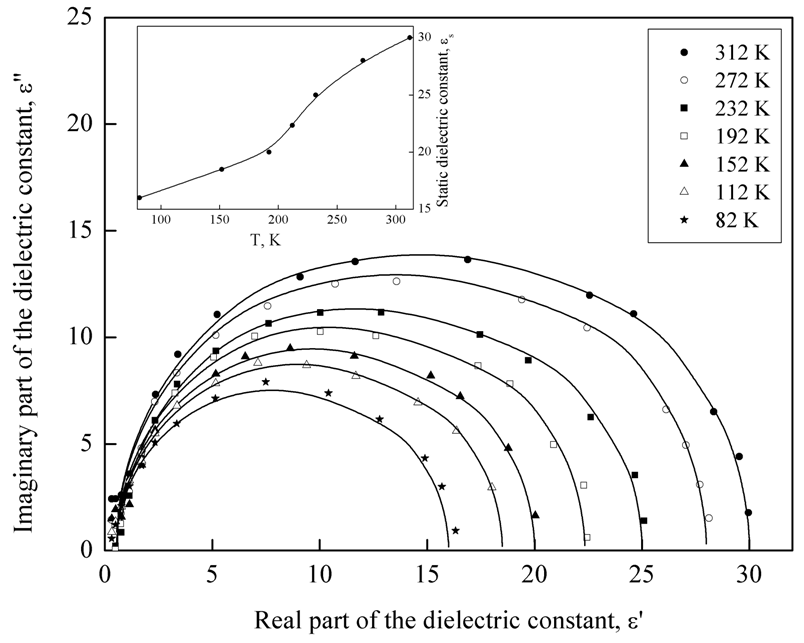Figure (8). Cole–Cole diagram for the investigated thin film samples at different temperatures. The solid curves drawn are the fitting of Cole–Cole equations to the calculated values of ε' and ε". The dependence of the calculated εs on the applied temperature are represented in the inset

### 4. Conclusions

A comprehensive electric and dielectric study on the effect of doping of a-Se thin films with 0.008 at. wt.% of Dy in the temperature range 312–82 K and frequency range 50 Hz–80 kHz allows us to draw the following conclusions:
(1) A graphical relation between ac conductivity and temperature illustrated a strong temperature dependence and small frequency dependence (f ≤ 40 kHz) for σac in the region of high temperature (312 K ≥ T >152 K). While for f > 40 kHz, σac depends mainly on the applied frequency and this dependence decreases as frequency increases.
(2) The calculated values of the power exponent s showed decreases from high to low values with increasing temperature.
(3) Among the different calculated ac conduction models, the CBH model is fitted fairly well with the experimental data of the function σac = f(T) in the low temperature range (82-187 K) with a deviation in the high temperature range.
(4) The calculated values of normal and inverted MN parameters (and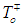) are used to fit the measured electrical conductivities of investigated film samples. A reasonable fitting appears in the high temperature region (187-312 K) with a deviation in the low temperature range.
(5) The arc shape of the Cole–Cole diagram leads to one value for the optical dielectric constant,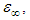and different values for the static dielectric constant εs, throughout the temperature range, 312–82 K.

### References

  Z. Yang, W. Chen and L. Luo, Dy3+-doped Ge-Ga-Sb-Se glasses for 1.3 μm optical fiber amplifiers, J. Non-Cryst. Solids 351 (2005) 2513-2518.  J. Heo, 1.3 μm-emission properties and local structure of Dy3+ in chalcohalide glasses, C. R. Chimie. 5 (2002) 739-749.  D.W. Hewak, B.N. Samson, J.A. Medeiros Neto, R.I. Laming and D.N. Payne, Emission at 1.3 μm from dysprosium-doped Ga:La:S glass, Electron Lett. 30 (1994) 968-970.  K. Wei, D.P. Machewirth, J. Wenzel, E. Snitzer and G.H. Sigel, Jr, Spectroscopy of Dy3+ in Ge-Ga-S glass and its suitability for 1.3 μm fiber-optical amplifier applications, Opt. Lett. 19 (1994) 904-906.  G. Tang, Z. Yang, L. Luo and W. Chen, Dy3+-doped chalcohalide glass for 1.3-µm optical fiber amplifiers, J. Mater. Res. 23 (2008) 954-961.  N.F. Mott and E.A. Davis, Electronic Processes in Non-Crystalline Materials, 2nd edn Clarendon Press, Oxford, 1979.  S.R. Elliott, Physics of Amorphous Materials, 2nd edn Longmann Scientific & Technical, New York, 1990.  A. Zakery and S. R. Elliott, Optical Nonlinearities in chalcogenide glasses and their applications, Springer Berlin Heidelberg, 2007.  B.J. Park, H. Seak, J.T. Ahn, Y.G. Chol, J. Heo and W.T. Chung, Dy3+ doped Ge-Ga-Sb-Se glasses and optical fiber for the mid-IR gain media, J. Ceram. Soc. Japan. 116 (2008) 1087-1091.  J. Heo, Rare-earth doped chalcogenide glasses for fiber-optic amplifiers, J. Non-Cryst. Solids 326 & 327 (2003) 410-415.  Z.Y. Shen, Y.M. Li, Q.G. Hu, W.Q. Luo and Z.M. Wang, Dielectric properties of B–site charge balanced Dy–doped SrTiO ceramics for energy storage, J. Electroceram. 34 (2015) 236-240.  M.F. Kotkata, M.S. AL-Kotb and F.A. Abdel-wahab, Doping effect of Sm on the energy gap and optical dispersion of a-Se, Chalcogenide Letters 7 (2010) 145-157.  E.R. Barney, Z. Tang, A. Seddon, D. Furniss, S. Sujecki, T. Benson, N. Neate and D. Gianolio, The local environment of Dy3+ in selenium-rich chalcogenide glasses, RSC Adv. 4 (2014) 42364-42371.  A. Ghosh, Frequency-dependent conductivity in bismuth-vanadate glassy semiconductors, Phys. Rev. B 41 (1990) 1479-1488.  A.K. Jonscher, Alternating current diagnostics of poorly conducting thin films, Thin Solid Films 36 (1976) 1-20.  A.K. Jonscher, The universal dielectric response, Nature 267 (1977) 673-679.  R. Long, Frequency-dependent loss in amorphous semiconductors, Adv. Phys. 31 (1982) 553-637.  S.R. Elliot, A.c. conduction in amorphous chalcogenide and pnictide semiconductors, Adv. Phys. 36 (1987) 135-218.  S.R. Eilliot, A theory of a.c. conduction in chalcogenide glasses, Phil. Mag. B 36 (1977) 1291-1304.  A. Ghosh, Transport properties of Vanadium Germenate glassy semiconductor, Phys. Rev. B 42 (1990) 5665-5676.  K. Shimakawa and F.A. Abdel Wahab, The Meyer–Neldel rule in chalcogenide glasses, Appl. Phys. Lett. 70 (1997) 652-654.  F.A. Abdel Wahab, Signature of the Meyer–Neldel rule on the correlated barrier-hopping model, J. Appl. Phys. 91 (2002) 265-270.  W. Meyer and H. Neldel, Relation between the energy constant and the quantity constant in the conductivity-temperature formula of oxide semiconductors, Z. Tech. Phys. 12 (1937) 588-593.  F.A. Abdel Wahab, Unified hopping model for dc and ac conduction in chalcogenide glasses Philos. Mag. B 82 (2002) 1327-1333.  T.A. Abtew, M.L. Zhang, Y. Pan and D.A. Drabold, Electrical conductivity and Meyer–Neldel rule: The role of localized states in hydrogenated amorphous silicon, J. Non-Cryst. Solids 354 (2008) 2909–2913.  M. Fox, Optical Properties of Solids, 2nd edn Oxford university press, New York, (2010).  P. Debye, Polar Molecules, Dover, New York, 1929.  R.H. Cole and K.S. Cole, Dispersion and Absorption in Dielectrics I. Alternating Current Characteristics, J. Chem. Phys. 9 (1941) 341-351.